# RS Aggarwal Solutions for Class 8 Maths Chapter 11 - Compound Interest Exercise 11B

Learners can view and download the RS Aggarwal Solutions for the Exercise 11B of Class 8 Maths Chapter 11, Compound Interest from the following link. BYJU’S expert team has designed solutions efficiently, and understandably that helps students solve problems in the most efficient possible ways. This exercise contains 30 questions; all are based on compound interest. By practising regularly, they can excel in board exams without fail. By the RS Aggarwal Solutions for class 8, students will be able to grasp the concepts entirely.

## Download PDF of RS Aggarwal Solutions for Class 8 Maths Chapter 11 – Compound Interest Exercise 11B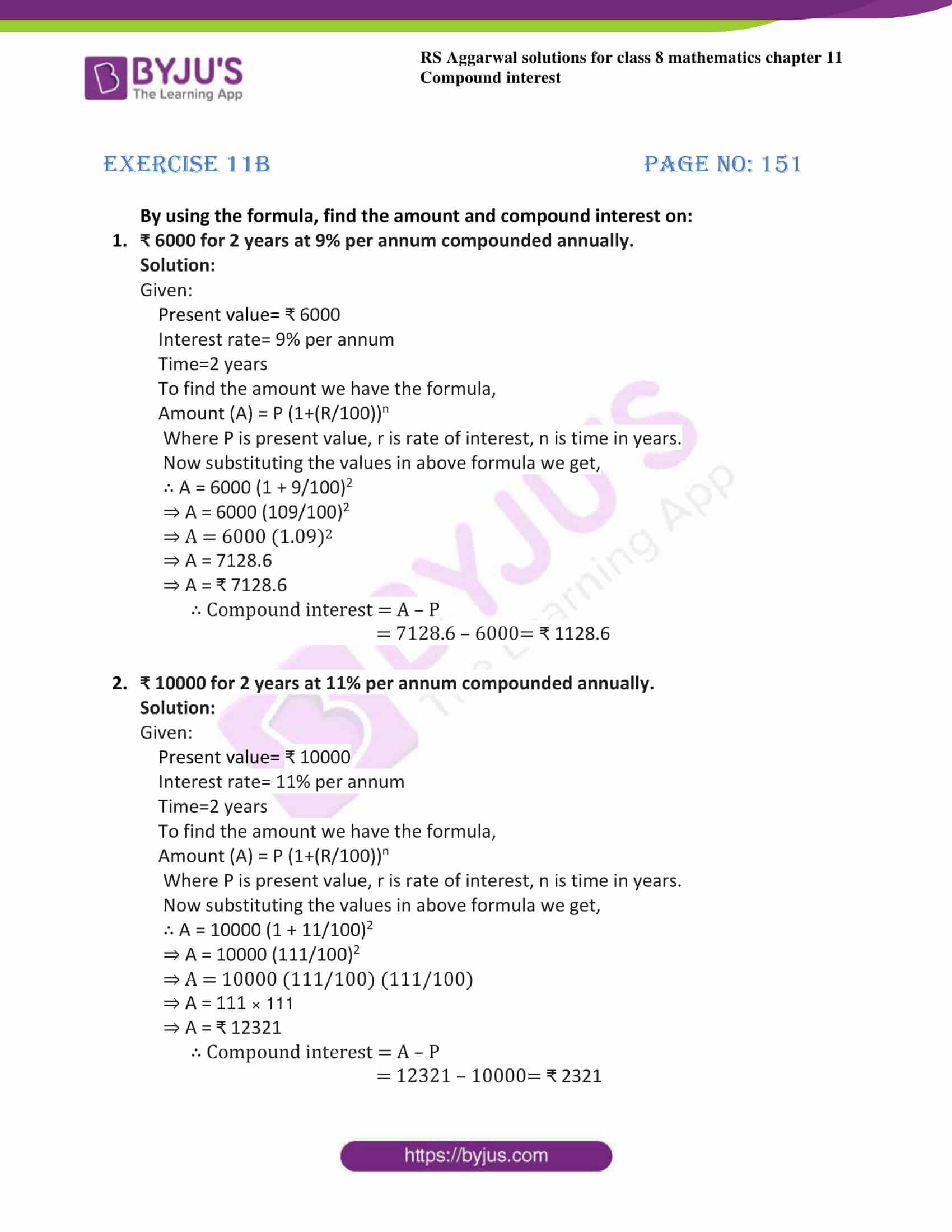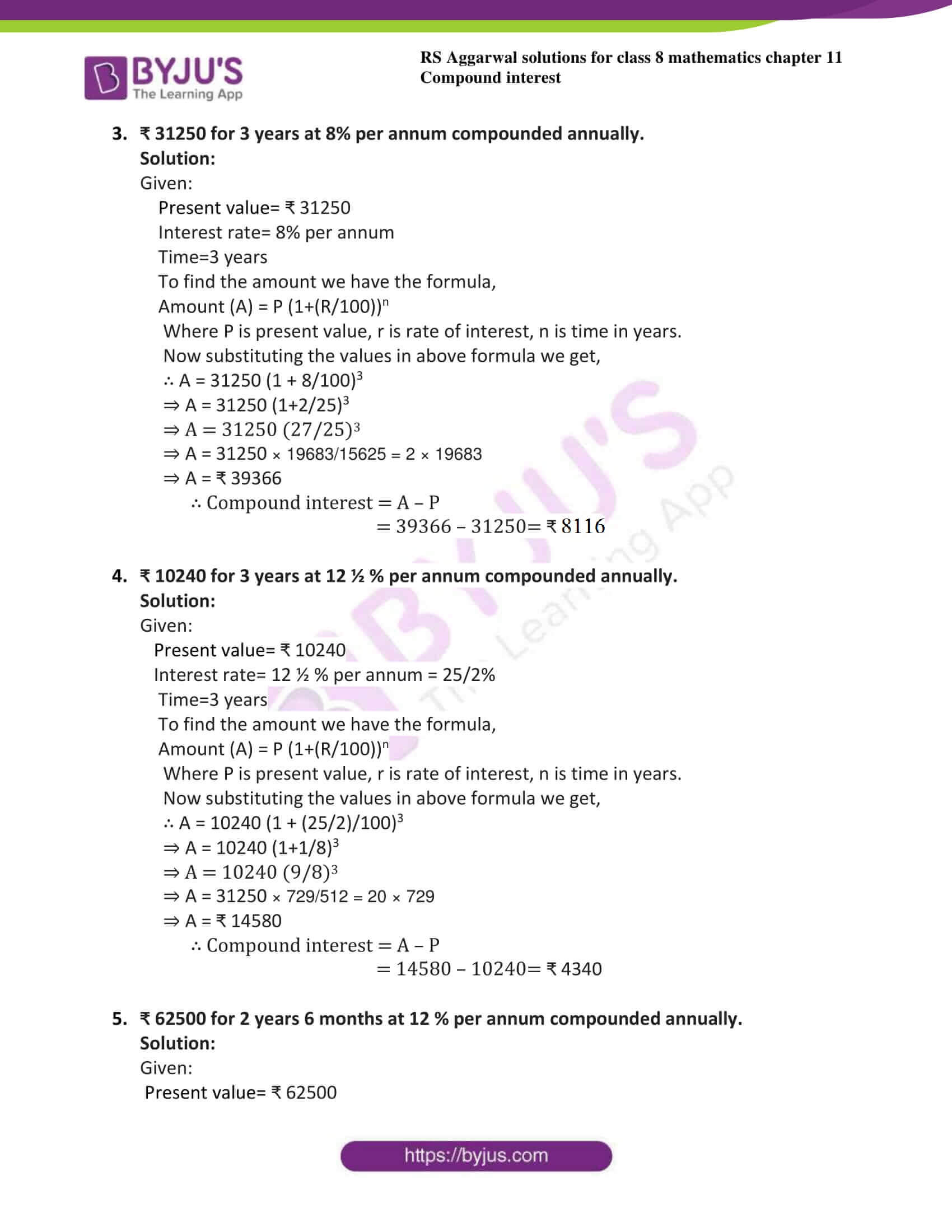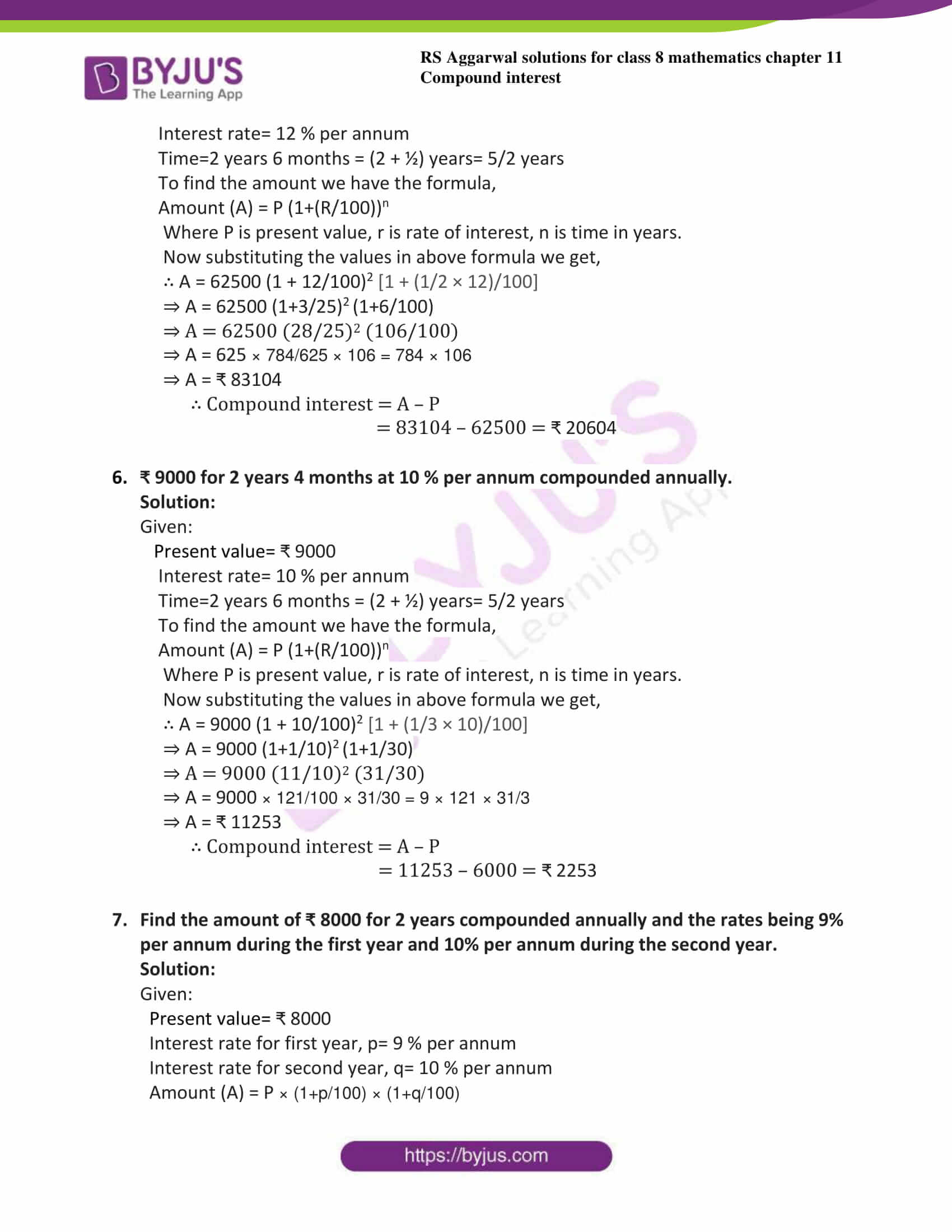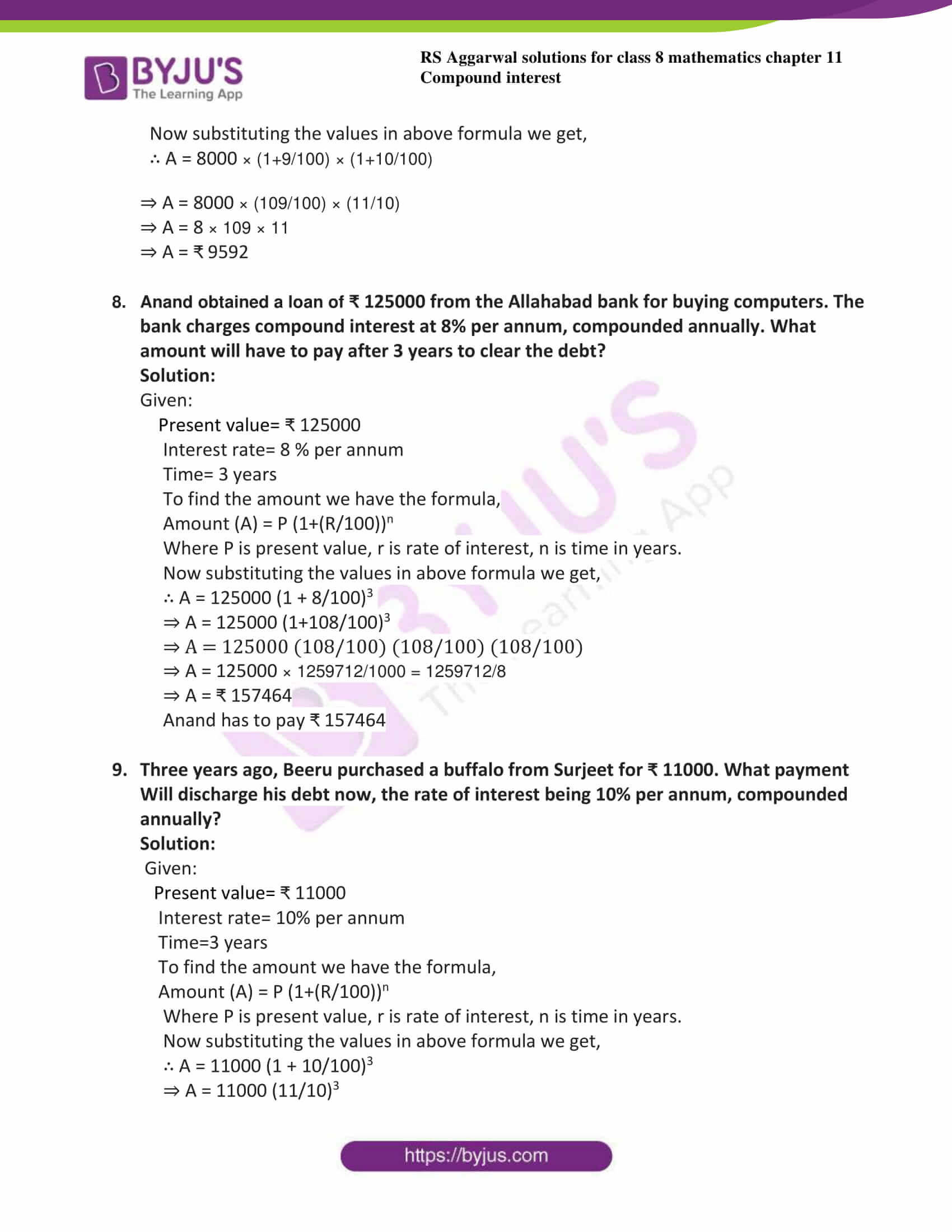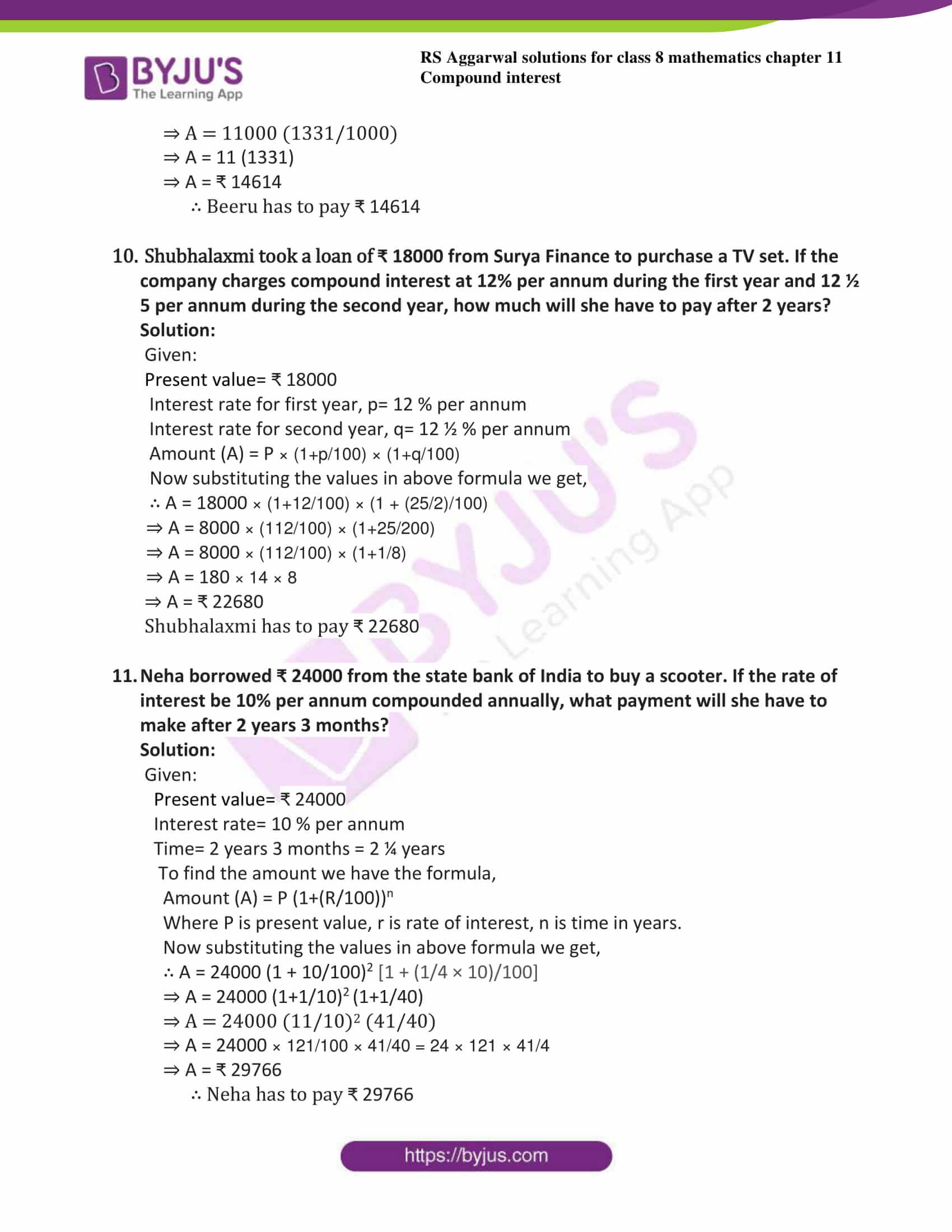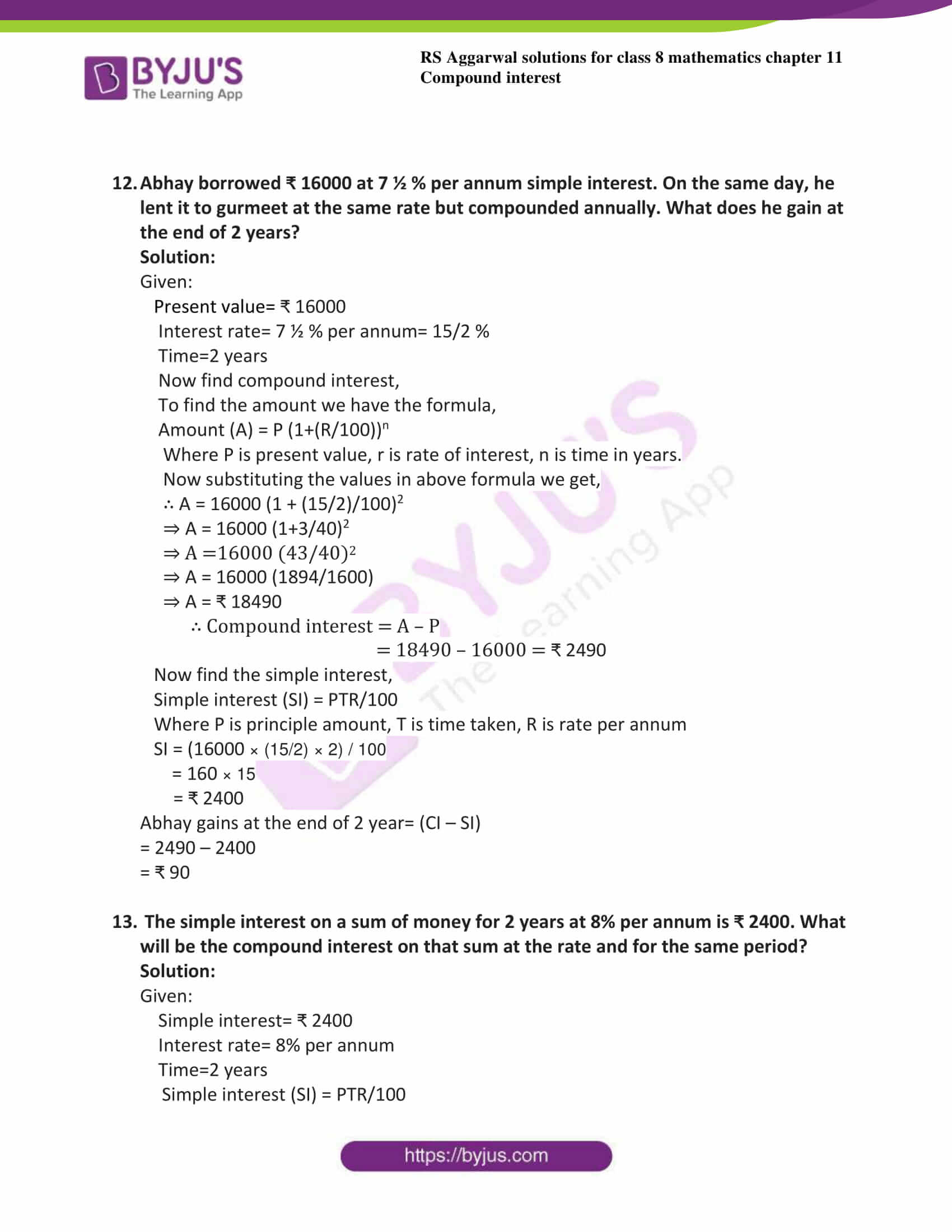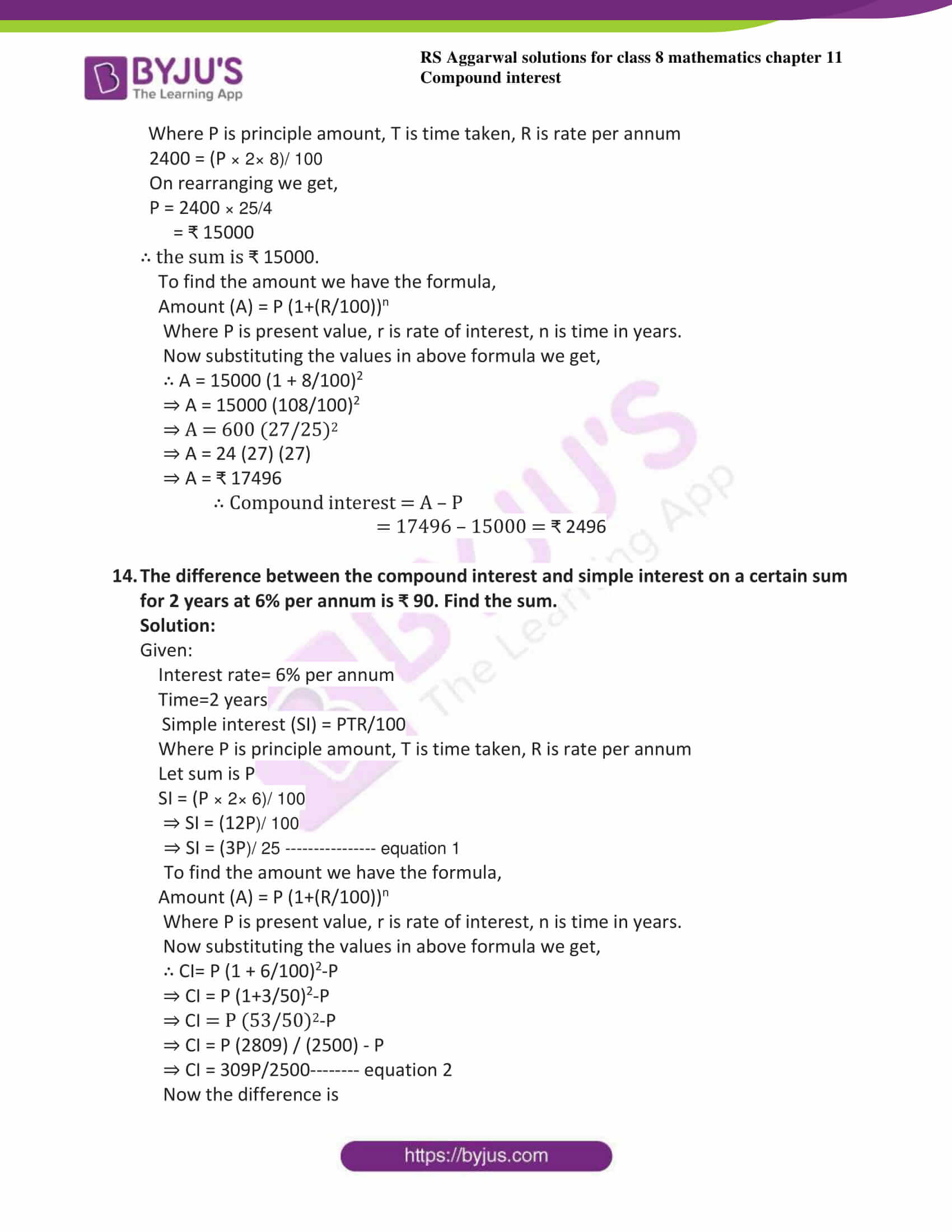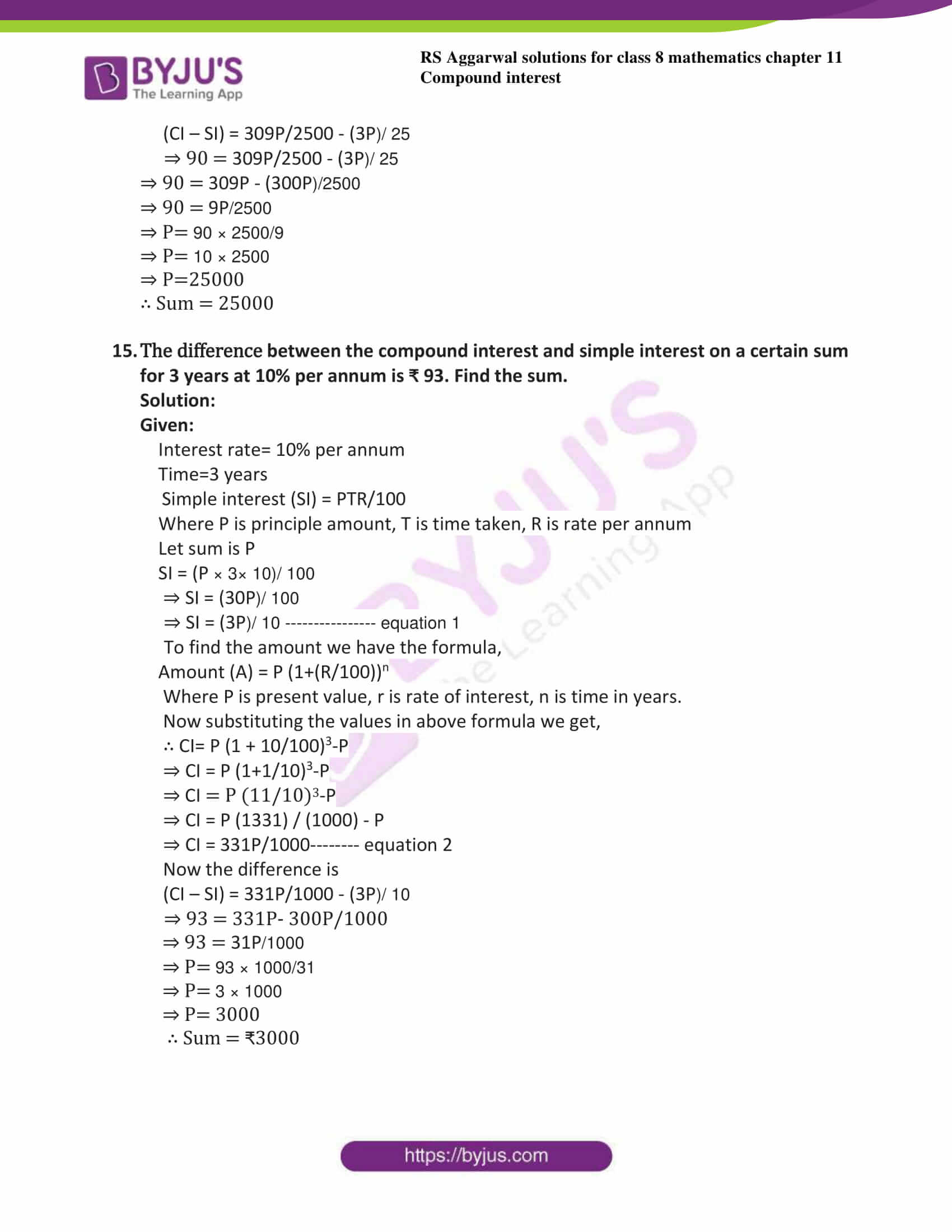### Access answers to Maths RS Aggarwal Solutions for Class 8 Chapter 11 – Compound Interest Exercise 11B

By using the formula, find the amount and compound interest on:

1. ₹ 6000 for 2 years at 9% per annum compounded annually.

Solution:

Given:

Present value= ₹ 6000

Interest rate= 9% per annum

Time=2 years

To find the amount we have the formula,

Amount (A) = P (1+(R/100))n

Where P is present value, r is rate of interest, n is time in years.

Now substituting the values in above formula we get,

∴ A = 6000 (1 + 9/100)2

⇒ A = 6000 (109/100)2

⇒ A = 6000 (1.09)2

⇒ A = 7128.6

⇒ A = ₹ 7128.6

∴ Compound interest = A – P

= 7128.6 – 6000= ₹ 1128.6

2. ₹ 10000 for 2 years at 11% per annum compounded annually.

Solution:

Given:

Present value= ₹ 10000

Interest rate= 11% per annum

Time=2 years

To find the amount we have the formula,

Amount (A) = P (1+(R/100))n

Where P is present value, r is rate of interest, n is time in years.

Now substituting the values in above formula we get,

∴ A = 10000 (1 + 11/100)2

⇒ A = 10000 (111/100)2

⇒ A = 10000 (111/100) (111/100)

⇒ A = 111 × 111

⇒ A = ₹ 12321

∴ Compound interest = A – P

= 12321 – 10000= ₹ 2321

3. ₹ 31250 for 3 years at 8% per annum compounded annually.

Solution:

Given:

Present value= ₹ 31250

Interest rate= 8% per annum

Time=3 years

To find the amount we have the formula,

Amount (A) = P (1+(R/100))n

Where P is present value, r is rate of interest, n is time in years.

Now substituting the values in above formula we get,

∴ A = 31250 (1 + 8/100)3

⇒ A = 31250 (1+2/25)3

⇒ A = 31250 (27/25)3

⇒ A = 31250 × 19683/15625 = 2 × 19683

⇒ A = ₹ 39366

∴ Compound interest = A – P

= 39366 – 31250= ₹ 8116

4. ₹ 10240 for 3 years at 12 ½ % per annum compounded annually.

Solution:

Given:

Present value= ₹ 10240

Interest rate= 12 ½ % per annum = 25/2%

Time=3 years

To find the amount we have the formula,

Amount (A) = P (1+(R/100))n

Where P is present value, r is rate of interest, n is time in years.

Now substituting the values in above formula we get,

∴ A = 10240 (1 + (25/2)/100)3

⇒ A = 10240 (1+1/8)3

⇒ A = 10240 (9/8)3

⇒ A = 31250 × 729/512 = 20 × 729

⇒ A = ₹ 14580

∴ Compound interest = A – P

= 14580 – 10240= ₹ 4340

5. ₹ 62500 for 2 years 6 months at 12 % per annum compounded annually.

Solution:

Given:

Present value= ₹ 62500

Interest rate= 12 % per annum

Time=2 years 6 months = (2 + ½) years= 5/2 years

To find the amount we have the formula,

Amount (A) = P (1+(R/100))n

Where P is present value, r is rate of interest, n is time in years.

Now substituting the values in above formula we get,

∴ A = 62500 (1 + 12/100)2 [1 + (1/2 × 12)/100]

⇒ A = 62500 (1+3/25)2 (1+6/100)

⇒ A = 62500 (28/25)2 (106/100)

⇒ A = 625 × 784/625 × 106 = 784 × 106

⇒ A = ₹ 83104

∴ Compound interest = A – P

= 83104 – 62500 = ₹ 20604

6. ₹ 9000 for 2 years 4 months at 10 % per annum compounded annually.

Solution:

Given:

Present value= ₹ 9000

Interest rate= 10 % per annum

Time=2 years 6 months = (2 + ½) years= 5/2 years

To find the amount we have the formula,

Amount (A) = P (1+(R/100))n

Where P is present value, r is rate of interest, n is time in years.

Now substituting the values in above formula we get,

∴ A = 9000 (1 + 10/100)2 [1 + (1/3 × 10)/100]

⇒ A = 9000 (1+1/10)2 (1+1/30)

⇒ A = 9000 (11/10)2 (31/30)

⇒ A = 9000 × 121/100 × 31/30 = 9 × 121 × 31/3

⇒ A = ₹ 11253

∴ Compound interest = A – P

= 11253 – 6000 = ₹ 2253

7. Find the amount of ₹ 8000 for 2 years compounded annually and the rates being 9% per annum during the first year and 10% per annum during the second year.

Solution:

Given:

Present value= ₹ 8000

Interest rate for first year, p= 9 % per annum

Interest rate for second year, q= 10 % per annum

Amount (A) = P × (1+p/100) × (1+q/100)

Now substituting the values in above formula we get,

∴ A = 8000 × (1+9/100) × (1+10/100)

⇒ A = 8000 × (109/100) × (11/10)

⇒ A = 8 × 109 × 11

⇒ A = ₹ 9592

8. Anand obtained a loan of ₹ 125000 from the Allahabad bank for buying computers. The bank charges compound interest at 8% per annum, compounded annually. What amount will have to pay after 3 years to clear the debt?

Solution:

Given:

Present value= ₹ 125000

Interest rate= 8 % per annum

Time= 3 years

To find the amount we have the formula,

Amount (A) = P (1+(R/100))n

Where P is present value, r is rate of interest, n is time in years.

Now substituting the values in above formula we get,

∴ A = 125000 (1 + 8/100)3

⇒ A = 125000 (1+108/100)3

⇒ A = 125000 (108/100) (108/100) (108/100)

⇒ A = 125000 × 1259712/1000 = 1259712/8

⇒ A = ₹ 157464

Anand has to pay ₹ 157464

9. Three years ago, Beeru purchased a buffalo from Surjeet for ₹ 11000. What payment

Will discharge his debt now, the rate of interest being 10% per annum, compounded annually?

Solution:

Given:

Present value= ₹ 11000

Interest rate= 10% per annum

Time=3 years

To find the amount we have the formula,

Amount (A) = P (1+(R/100))n

Where P is present value, r is rate of interest, n is time in years.

Now substituting the values in above formula we get,

∴ A = 11000 (1 + 10/100)3

⇒ A = 11000 (11/10)3

⇒ A = 11000 (1331/1000)

⇒ A = 11 (1331)

⇒ A = ₹ 14614

∴ Beeru has to pay ₹ 14614

10. Shubhalaxmi took a loan of ₹ 18000 from Surya Finance to purchase a TV set. If the company charges compound interest at 12% per annum during the first year and 12 ½ 5 per annum during the second year, how much will she have to pay after 2 years?

Solution:

Given:

Present value= ₹ 18000

Interest rate for first year, p= 12 % per annum

Interest rate for second year, q= 12 ½ % per annum

Amount (A) = P × (1+p/100) × (1+q/100)

Now substituting the values in above formula we get,

∴ A = 18000 × (1+12/100) × (1 + (25/2)/100)

⇒ A = 8000 × (112/100) × (1+25/200)

⇒ A = 8000 × (112/100) × (1+1/8)

⇒ A = 180 × 14 × 8

⇒ A = ₹ 22680

Shubhalaxmi has to pay ₹ 22680

11. Neha borrowed ₹ 24000 from the state bank of India to buy a scooter. If the rate of interest be 10% per annum compounded annually, what payment will she have to make after 2 years 3 months?

Solution:

Given:

Present value= ₹ 24000

Interest rate= 10 % per annum

Time= 2 years 3 months = 2 ¼ years

To find the amount we have the formula,

Amount (A) = P (1+(R/100))n

Where P is present value, r is rate of interest, n is time in years.

Now substituting the values in above formula we get,

∴ A = 24000 (1 + 10/100)2 [1 + (1/4 × 10)/100]

⇒ A = 24000 (1+1/10)2 (1+1/40)

⇒ A = 24000 (11/10)2 (41/40)

⇒ A = 24000 × 121/100 × 41/40 = 24 × 121 × 41/4

⇒ A = ₹ 29766

∴ Neha has to pay ₹ 29766

12. Abhay borrowed ₹ 16000 at 7 ½ % per annum simple interest. On the same day, he lent it to gurmeet at the same rate but compounded annually. What does he gain at the end of 2 years?

Solution:

Given:

Present value= ₹ 16000

Interest rate= 7 ½ % per annum= 15/2 %

Time=2 years

Now find compound interest,

To find the amount we have the formula,

Amount (A) = P (1+(R/100))n

Where P is present value, r is rate of interest, n is time in years.

Now substituting the values in above formula we get,

∴ A = 16000 (1 + (15/2)/100)2

⇒ A = 16000 (1+3/40)2

⇒ A =16000 (43/40)2

⇒ A = 16000 (1894/1600)

⇒ A = ₹ 18490

∴ Compound interest = A – P

= 18490 – 16000 = ₹ 2490

Now find the simple interest,

Simple interest (SI) = PTR/100

Where P is principle amount, T is time taken, R is rate per annum

SI = (16000 × (15/2) × 2) / 100

= 160 × 15

= ₹ 2400

Abhay gains at the end of 2 year= (CI – SI)

= 2490 – 2400

= ₹ 90

13. The simple interest on a sum of money for 2 years at 8% per annum is ₹ 2400. What will be the compound interest on that sum at the rate and for the same period?

Solution:

Given:

Simple interest= ₹ 2400

Interest rate= 8% per annum

Time=2 years

Simple interest (SI) = PTR/100

Where P is principle amount, T is time taken, R is rate per annum

2400 = (P × 2× 8)/ 100

On rearranging we get,

P = 2400 × 25/4

= ₹ 15000

∴ the sum is ₹ 15000.

To find the amount we have the formula,

Amount (A) = P (1+(R/100))n

Where P is present value, r is rate of interest, n is time in years.

Now substituting the values in above formula we get,

∴ A = 15000 (1 + 8/100)2

⇒ A = 15000 (108/100)2

⇒ A = 600 (27/25)2

⇒ A = 24 (27) (27)

⇒ A = ₹ 17496

∴ Compound interest = A – P

= 17496 – 15000 = ₹ 2496

14. The difference between the compound interest and simple interest on a certain sum for 2 years at 6% per annum is ₹ 90. Find the sum.

Solution:

Given:

Interest rate= 6% per annum

Time=2 years

Simple interest (SI) = PTR/100

Where P is principle amount, T is time taken, R is rate per annum

Let sum is P

SI = (P × 2× 6)/ 100

⇒ SI = (12P)/ 100

⇒ SI = (3P)/ 25 —————- equation 1

To find the amount we have the formula,

Amount (A) = P (1+(R/100))n

Where P is present value, r is rate of interest, n is time in years.

Now substituting the values in above formula we get,

∴ CI= P (1 + 6/100)2-P

⇒ CI = P (1+3/50)2-P

⇒ CI = P (53/50)2-P

⇒ CI = P (2809) / (2500) – P

⇒ CI = 309P/2500——– equation 2

Now the difference is

(CI – SI) = 309P/2500 – (3P)/ 25

⇒ 90 = 309P/2500 – (3P)/ 25

⇒ 90 = 309P – (300P)/2500

⇒ 90 = 9P/2500

⇒ P= 90 × 2500/9

⇒ P= 10 × 2500

⇒ P=25000

∴ Sum = 25000

15. The difference between the compound interest and simple interest on a certain sum for 3 years at 10% per annum is ₹ 93. Find the sum.

Solution:

Given:

Interest rate= 10% per annum

Time=3 years

Simple interest (SI) = PTR/100

Where P is principle amount, T is time taken, R is rate per annum

Let sum is P

SI = (P × 3× 10)/ 100

⇒ SI = (30P)/ 100

⇒ SI = (3P)/ 10 —————- equation 1

To find the amount we have the formula,

Amount (A) = P (1+(R/100))n

Where P is present value, r is rate of interest, n is time in years.

Now substituting the values in above formula we get,

∴ CI= P (1 + 10/100)3-P

⇒ CI = P (1+1/10)3-P

⇒ CI = P (11/10)3-P

⇒ CI = P (1331) / (1000) – P

⇒ CI = 331P/1000——– equation 2

Now the difference is

(CI – SI) = 331P/1000 – (3P)/ 10

⇒ 93 = 331P- 300P/1000

⇒ 93 = 31P/1000

⇒ P= 93 × 1000/31

⇒ P= 3 × 1000

⇒ P= 3000

∴ Sum = ₹3000

## RS Aggarwal Solutions for Class 8 Maths Chapter 11 – Compound Interest Exercise 11B

Exercise 11B of RS Aggarwal Solutions for Chapter 11, Compound Interest deals with the topics related to compound interest and methods of solving compound interest using the formulae. Some of the topics focused prior to exercise 11B include the following.

• Calculating compound interest by using formulae
• Applications of compound interest formula

The RS Aggarwal Solutions can help the students in practising and learning each and every concept as it provides solutions to all questions asked in the RS Aggarwal textbook.